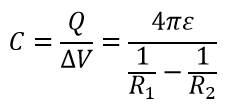# Capacitance of Concentric Spheres Calculator

The capacitance for spherical conductors can be obtained by evaluating the voltage difference between the conductors for a given charge on each. This voltage difference can be calculated by integrating the electric field along the radius between R1 and R2.

#### Result

F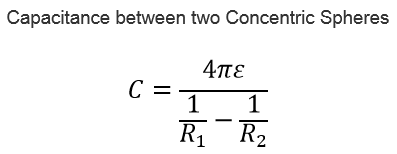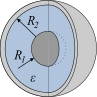Where,

R1 = Radius of inner Sphere

R2 = Radius of outer Sphere

ε=εo εr

εo=8.854187817e-12

εr=Relative Permittivity

#### The Derivation of this Capacitance is shown below:

The capacitance for spherical conductors can be obtained by evaluating the voltage difference between the conductors for a given charge on each. This voltage difference can be calculated by integrating the electric field along the radius between R1 and R2.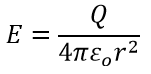The voltage difference can be calculated by integrating the electric field along the radius between R1 and R2.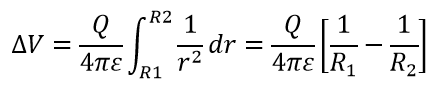The capacitance can be calculated as a function of the charge and the voltage difference between the spheres.